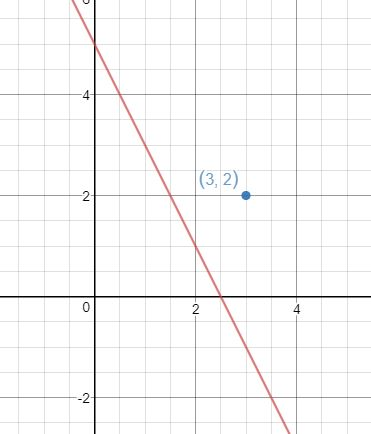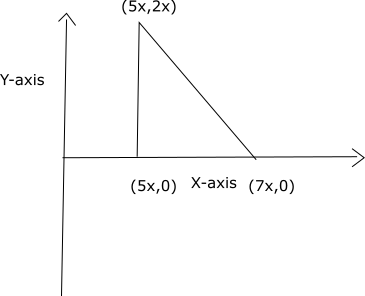# CAT Questions on Coordinate Geometry

0
3170

## CAT Questions and answers on coordinate geometry:

CAT Mocks One time Offer: 25 MBA Mocks + 30 CAT sectionals at just Rs. 1249

Question 1:

What is the reflection of the point (4 , -3) in the line y = 1?

a) (4, -5)
b) (4, 5)
c) (-4, -5)
d) (-4, 5)

Question 2:

At what point does the line 3x + 2y = -12 intercept the Y-axis?

a) (0,6)
b) (0,-6)
c) (-4,0)
d) (4,0)

Question 3:

The area of triangle with vertices (5x,2x), (7x, 0) and (5x, 0) is 72 sq units. If x is a positive integer, what is the value of x?

a) 6
b) 3
c) 9
d) 12
e)15

Question 4:

Find the intercepts made by the line 7x + 6y – 42 = 0 on y and x axis respectively.

a) (6,7)
b) (3,2)
c) (2,3)
d) (7,6)

Question 5:

If the coordinates of the point that is a reflection of (3,2) with respect to 2x+y=5 are (a,b), then find the value of (a+b-0.4).

Solution:Line perpendicular to 2x+y=5 has a slope of 1/2.
Line passing through (3,2) with a slope of 1/2 => y-2 = 1/2(x-3)
2y-4 = x-3 => x-2y = -1
Intersection point = (9/5,7/5)
Reflection point = (a,b)

(9/5, 7/5) is the midpoint of (3,2) and (a,b). Hence, by midpoint formula
(a+3)/2 = 9/5 => a = 3/5
(b+2)/2 = 7/5 => b = 4/5
a+b-0.4 = 1.4 – 0.4 = 1

Solutions (1 to 4)

Reflection of point (x,y) in line y=a is (x,-y+2a)
Now, Reflection of point (4,-3) in line y=1
= [4,3+2(1)]=(4,5)
=> Ans – (B)

CAT Mocks One time Offer: 25 MBA Mocks + 30 CAT sectionals at just Rs. 1249

The line 3x + 2y = -12 will intercept the y-axis at x = 0
Thus, substituting value of x in above equation
=> 3(0) + 2y = -12
=> y = -12/2 = -6
Thus, the line will intercept y axis at (0,-6)
=> Ans – (B)

The diagram with the given vertices is given below:ABC is a right angled triangle with AB = BC = 2x and right angles at $$\angle ABC$$
Thus, area of the triangle = 1/2 * AB * BC = 1/2 * 2x * 2x = $$2x^2$$ = 72
=> x = $$\pm$$6
Since x is positive, x = 6